# CONCAT function in Excel to concatenate text strings

The tutorial describes some common uses of the CONCAT function and explains how it differs from its predecessor - the CONCATENATE function.

Traditionally, string concatenation in Excel is done with the help of the CONCATENATE function or the ampersand symbol. In modern Excel, these old-school methods give way to CONCAT - a newer and improved function to combine text from multiple cells into one cell.

## Excel CONCAT function

The CONCAT function in Excel is used to combine strings from multiple cells or ranges into a single cell.

It is categorized under Text functions and is available in Excel for Microsoft 365, Excel 2021, Excel 2019, and Excel for the web.

The syntax is as simple as this:

CONCAT(text1, [text2],…)

Where text1 (required) and text2 (optional) are the strings to be joined.

Arguments can be supplied as text values, cell or range references. A maximum of 255 text arguments are accepted. Strings are concatenated in the order they appear in a formula.

As an example, let's write a CONCAT formula in its simplest form to merge two text strings - user name and domain name. Please pay attention that strings supplied directly to the formula should always be enclosed in double quotation marks like this:

`=CONCAT("john", "@gmail.com")`

The below screenshot shows the concatenation result: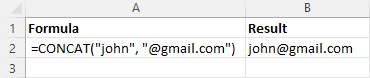### CONCAT function - 5 things to remember

Before we dive into practical examples, let me briefly outline a few things to take notice of:

1. The CONCAT function requires at least one text argument to work. If any of the arguments is invalid (e.g. represented by another function that throws an error), an error will occur.
2. The result of the CONCATENATE function is always a text string, even when you are combining numbers.
3. If the resulting string exceeds 32,767 characters, a #VALUE! error occurs.
4. Excel CONCAT doesn't have a predefined option for delimiter. To separate the combined text pieces, put delimiters between the concatenated strings like shown in this example.
5. Empty cells are ignored.

## How to use the CONCAT function in Excel - formula examples

Now comes the most interesting part - using the CONCAT function in different scenarios to handle real-life tasks.

### CONCAT two or more cells

For starters, let us discuss the basic use case - concatenating cells without separator. Obviously, you just need to use the corresponding cells references for the text1, text2, …, textn arguments.

For instance, to concatenate two cells (A2 and B2), the formula in C2 is:

`=CONCAT(A2, B2)`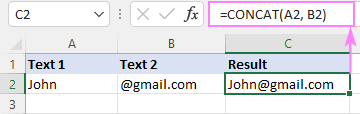To join strings from multiple contiguous cells, you can supply either individual cells refences or a single range reference.

For instance, to merge strings in cells A2 through C2, both of the below formulas will work:

`=CONCAT(A2, B2, C2)`

`=CONCAT(A2:C2)`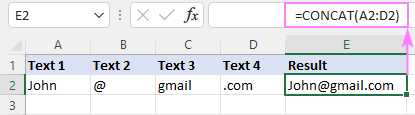If some of the combined cells are empty, do not worry - the CONCAT function automatically ignores blank cells.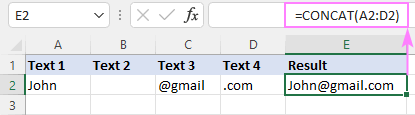### CONCAT two or more columns

To concatenate columns row by row, this is what you need to do:

1. Write a CONCAT formula to combine cells in the first row.
2. Copy the formula down to as many rows as needed.

For example, to combine strings in columns A, B, C in each row, enter this formula in D2:

`=CONCAT(A2:C2)`

And then, drag the fill handle down to copy the formula to the below rows. If there are no gaps in the source data, you can just double-click the fill handle. For the detailed instructions, please see How to copy formula in Excel.

Due to the use of relative references, the formula will adjust appropriately for each row. That is, in D3, the reference will change to A3:C3, in D4 to A4:C4, and so on.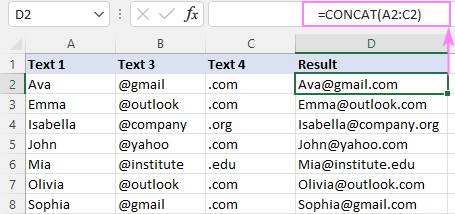### Concatenate a range of cells

Concatenating a range of cells in Excel used to be a challenge. But not any longer! Now, you can simply put a range reference in the text argument of the CONCAT function:

`=CONCAT(A2:C3)`

As the result, all the values from the specified range are combined from left to right and then down to the next row: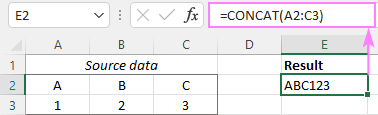In a similar manner, you can merge two or more non-adjacent ranges:

`=CONCAT(A2:C3, A5:C6)`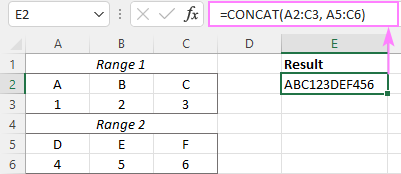### Concatenate all cells in column

To join values from all the cells in a specific column, serve a column reference to CONCAT. For example:

`=CONCAT(A:A)`

As the function skips empty cells, there will be no gaps in the resulting string: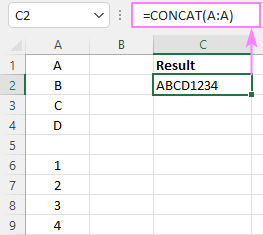To concatenate all cells in column except the header, use a range reference like A2:A1048576, where A2 is the first cell with data and A1048576 is the last cell in the column:

`=CONCAT(A2:A1048576)`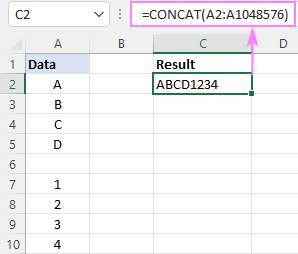Note. When concatenating all cells in a column, please be aware that Excel has a limit to the total number of characters a cell can contain - up to 32,767 characters. If the resulting string exceeds this limit, your formula will throw a #VALUE! error.

### CONCAT with separator (comma, space, line break, etc.)

As already mentioned, the syntax of the CONCAT function has no option for delimiter. However, that does not mean you cannot include it directly in a formula.

To concatenate strings with comma, put one of the following separators between the text arguments:

• Comma without space: ","
• Comma and space: ", "

For example, to combine strings from cells A2, B2 and C2 separating the values with a comma and a space, here's the formula to use:

`=CONCAT(A2, ", ", B2, ", ", C2)`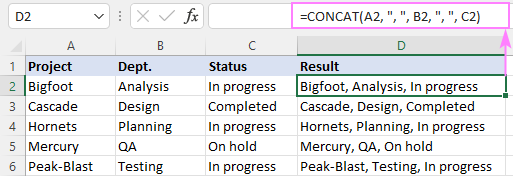To concatenate cells with spaces, put " " between the text arguments:

`=CONCAT(A2, " ", B2, " ", C2)`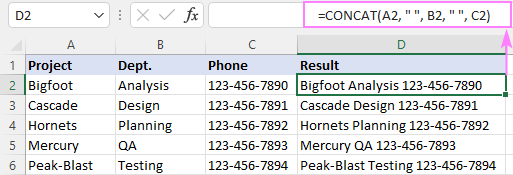To concatenate strings with line break, use CHAR(10), which is the ASCII code for line feed:

`=CONCAT(A2, CHAR(10), B2, CHAR(10), C2)`

For the results to display correctly, be sure to turn the Warp Text feature on.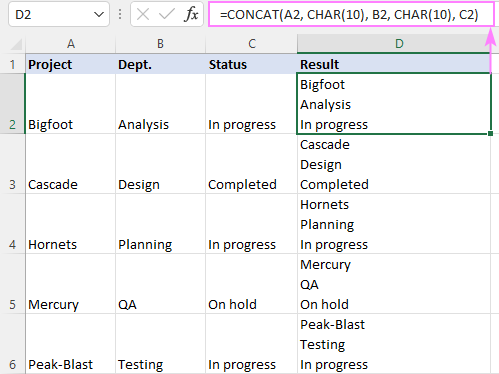At first sight, the CONCAT syntax not providing for a delimiter may look like a drawback. However, this enables you to use different delimiters within a single formula to combine multiple strings exactly the way you want!

For instance, you can insert a line break after text1 while separating text2 and text3 with a comma:

`=CONCAT(A2, CHAR(10), B2, ", ", C2)`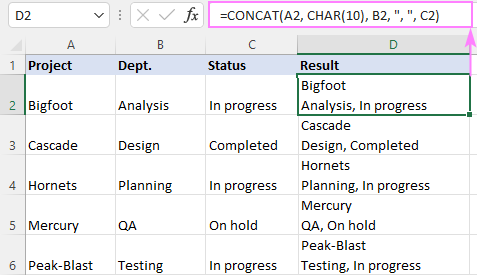Tip. To merge multiple values with a common separator, use the TEXTJOIN function.

### Concatenate dates

In situation when some of the combined values are dates, you may get confusing results like shown below:

`=CONCAT(A2, CHAR(10), B2)`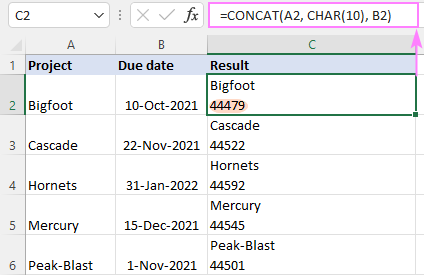What's wrong with that formula? Nothing. The CONCAT function does exactly what it is supposed to do - combines text strings. And any numeric values are converted to text values during the process. Because in the internal Excel system dates are stored as serial numbers, the CONCAT function uses those underlying numbers.

To have a date concatenated correctly, first convert it to a text strings with the help of the TEXT function, and then serve the string to CONCAT.

For example, to combine a text value in A2 and a date in B2, the formula is:

`=CONCAT(A2, CHAR(10), TEXT(B2, "d-mmm-yyyy"))`

Instead of "d-mmm-yyyy" you can use any other date format you like.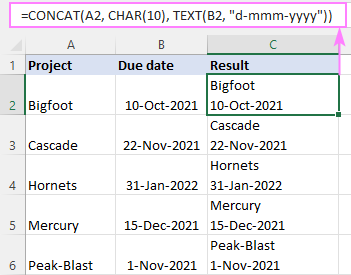### Concatenate text and another function

To concatenate strings produced by some other formulas, nest those formulas in the CONCAT function.

Suppose you wish to display the current time in your worksheet. The NOW function can do that with ease. To make it explicitly clear that it's the current time, you may want to add some explanatory text to the same cell.

To accomplish this task, we are concatenating the text "Current time: " and the NOW function. Additionally, we wrap NOW() inside TEXT to display time in the desired format. Without this refinement, time would appear as a decimal number that represents a date/time value internally in Excel.

`=CONCAT("Current time: ", TEXT(NOW(), "hh:mm AM/PM"))`

Because we've added a space character to the end of the text string, there's no need to include a separator in the formula - the result of concatenation looks perfect: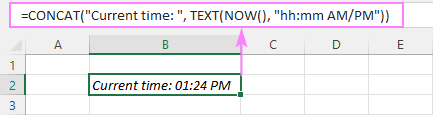## CONCAT vs. CONCATENATE in Excel

In fact, the CONCAT function is the replacement for CONCATENATE in new Excel versions. Though the older function is still supported for compatibility reasons, it is recommended to use the newer function instead.

From a technical viewpoint, there are two main differences between CONCAT and CONCATENATE in Excel:

1. First and foremost, the CONCAT function overcomes the biggest limitation of its predecessor - inability to handle a range of cells. Unlike the CONCATENATE function, CONCAT can combine all strings in a range saving you the trouble of specifying each cell reference individually.
2. The CONCAT function is only available in Excel 365, Excel 2021, Excel 2019 (Windows and Mac) and Excel Online. The CONCATENATE function is supported in all versions of Excel 2007 through 365.

In all other respects, the two functions are essentially the same.

That's how to use the CONCAT function in Excel to join text strings together. I thank you for reading and hope to see you on our blog next week!

1.Denis says: